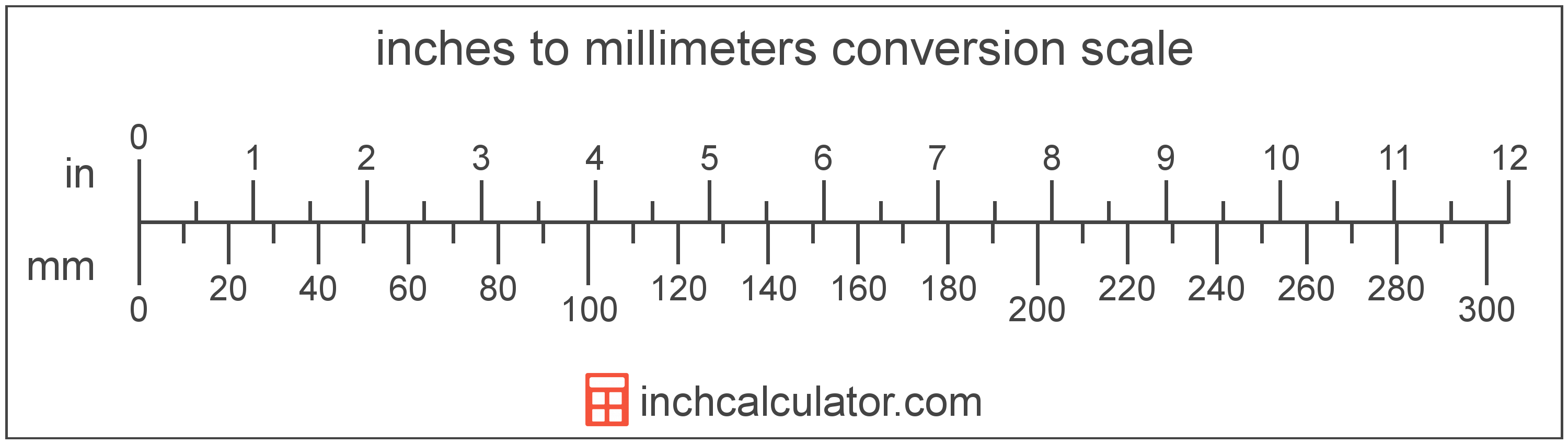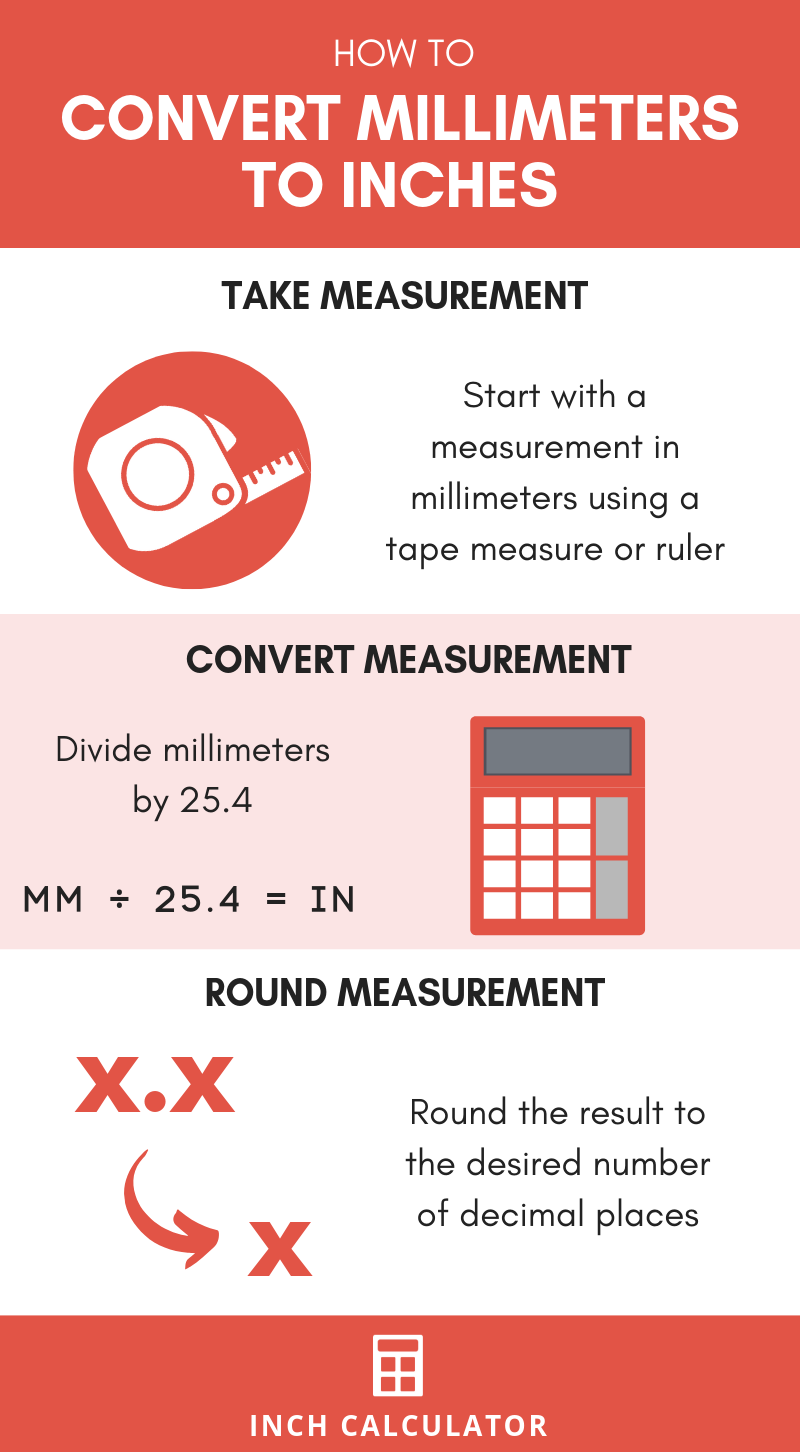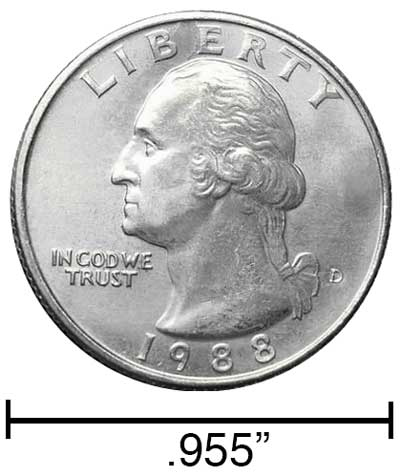# Millimeters to Inches Conversion Calculator

Enter the length in millimeters below to get the value converted to inches.

Results in Inches:1 mm = 0.03937 in
1 mm ≈ 3/64"
*inch fraction is rounded to nearest 1/64"
Do you want to convert inches to mm?

## How to Convert Millimeters to Inches

The fastest and easiest way to convert millimeters to inches (mm to in) is to use this simple formula:

inches = millimeters ÷ 25.4

Since there are 25.4 millimeters in one inch, we can calculate the length in inches by dividing the length in millimeters by 25.4. Thus, the formula to convert millimeters to inches is to divide the number of inches by 25.4.

For example, here's how to convert 50 millimeters to inches using the formula above.
inches = (50 mm ÷ 25.4) = 1.968504"### How to Convert Millimeters to Fractional Inches

You've learned how to convert millimeters to inches, but you might be wondering how to convert millimeters to fractional inches.

Start by converting mm to inches using the conversion formula above to get the result in decimal form, and then round to the nearest fractional inch. The number to the left of the decimal is the number of whole inches, and the numbers to the right of the decimal are what will be rounded to the nearest fractional inch.

To round, take the portion of the decimal result to the right of the decimal point and multiply it by the level of precision you want. You can choose to round to the nearest 1/16, 1/32, or 1/64 of an inch.

To round to the nearest 1/16 of an inch, for example, take the decimal portion and multiply it by 16. Then, round that result to the nearest whole number to get the numerator of the fractional inch measurement in 16ths of an inch.

For example, let's convert 100 mm to fractional inches with 1/16" precision following these steps.

Step 1: Divide 100 by 25.4 to convert mm to inches.

100 mm ÷ 25.4 = 3.937 in

Step 2: Multiply the decimal remainder by 16.

0.937 × 16 = 14.992

Step 3: Round this value to the nearest whole number; in this case, 14.992 can be rounded to 15. Place this value in a fraction over 16, so 15/16 is the remaining fraction.

Step 4: Put it all together by placing the whole number from the decimal form in front of the fraction.

100 mm ≈ 3 15/16"

Note that we now use the "approximately equal" sign above since we rounded, and thus 100 mm is not exactly equal to 3 and 15/16 inches.

Our inch fraction calculator can add millimeters and inches together, and it also automatically converts the results to US customary, imperial, and SI metric values.## What is a Millimeter?

One millimeter is equal to one-thousandth (1/1,000) of a meter, which is defined as the distance light travels in a vacuum in 1/299,792,458 of a second. One millimeter is equal to 0.0393701 inches.

The millimeter, or millimetre, is a multiple of the meter, which is the SI base unit for length. In the metric system, "milli" is the prefix for thousandths, or 10-3. Millimeters can be abbreviated as mm; for example, 1 millimeter can be written as 1 mm.

Millimeters are often represented by the smallest ticks on most metric rulers. To get a reference point of the size, the thickness of a US dime is 1.35mm.

## What is an Inch?

An inch is a unit of length equal to 1/12 of a foot or 1/36 of a yard. Because the international yard is legally defined to be equal to exactly 0.9144 meters, one inch is equal to 2.54 centimeters.

The inch is a US customary and imperial unit of length. Inches can be abbreviated as in; for example, 1 inch can be written as 1 in.

Inches can also be denoted using the symbol, otherwise known as a double-prime. Often a double-quote (") is used instead of a double-prime for convenience. A double-prime is commonly used to express 1 in as 1″.The standard ruler is 12 inches long and is a common tool for measuring a length in inches. Another frequently used tool to perform measurements in inches is a tape measure, which commonly comes in lengths from 6' - 35'. Other types of devices used to make measurements in inches include scales, calipers, measuring wheels, micrometers, yardsticks, and even lasers.

We recommend using a ruler or tape measure for measuring length, which can be found at a local retailer or home center. Rulers are available in imperial, metric, or a combination of both values, so make sure you get the correct type for your needs.

Need a ruler? Try our free downloadable and printable rulers, which include both imperial and metric measurements.

## mm to Inches Conversion Chart

Chart of millimeter measurements converted to inches and fractional inches rounded to the nearest 1/64"
Millimeters Inches (decimal) Inches (fraction)
1 mm 0.03937" 3/64"
2 mm 0.07874" 5/64"
3 mm 0.11811" 1/8"
4 mm 0.15748" 5/32"
5 mm 0.19685" 13/64"
6 mm 0.23622" 15/64"
7 mm 0.275591" 9/32"
8 mm 0.314961" 5/16"
9 mm 0.354331" 23/64"
10 mm 0.393701" 25/64"
11 mm 0.433071" 7/16"
12 mm 0.472441" 15/32"
13 mm 0.511811" 33/64"
14 mm 0.551181" 35/64"
15 mm 0.590551" 19/32"
16 mm 0.629921" 5/8"
17 mm 0.669291" 43/64"
18 mm 0.708661" 45/64"
19 mm 0.748031" 3/4"
20 mm 0.787402" 25/32"
21 mm 0.826772" 53/64"
22 mm 0.866142" 55/64"
23 mm 0.905512" 29/32"
24 mm 0.944882" 15/16"
25 mm 0.984252" 63/64"
26 mm 1.0236" 1 1/32"
27 mm 1.063" 1 1/16"
28 mm 1.1024" 1 7/64"
29 mm 1.1417" 1 9/64"
30 mm 1.1811" 1 3/16"
31 mm 1.2205" 1 7/32"
32 mm 1.2598" 1 17/64"
33 mm 1.2992" 1 19/64"
34 mm 1.3386" 1 11/32"
35 mm 1.378" 1 3/8"
36 mm 1.4173" 1 27/64"
37 mm 1.4567" 1 29/64"
38 mm 1.4961" 1 1/2"
39 mm 1.5354" 1 17/32"
40 mm 1.5748" 1 37/64"

## References

1. National Institute of Standards and Technology, SI Units - Length, https://www.nist.gov/pml/owm/si-units-length
2. United States Mint, Coin Specifications, https://www.usmint.gov/learn/coin-and-medal-programs/coin-specifications
3. National Institute of Standards and Technology, Checking the Net Contents of Packaged Goods, Handbook 133 - 2019 Edition, https://nvlpubs.nist.gov/nistpubs/hb/2019/NIST.HB.133-2019.pdf